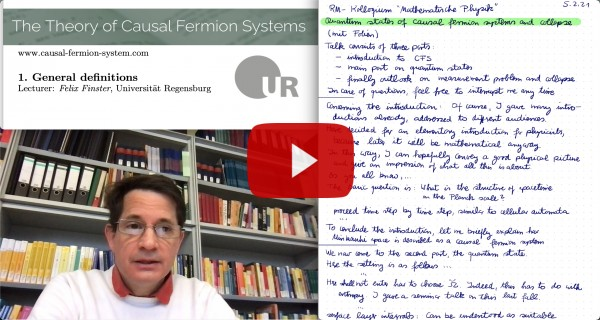# The Theory of Causal Fermion Systems

## The Euler-Lagrange Equations## The General Euler-Lagrange Equations

A minimizing measure $\rho$ of the causal action principle satisfies Euler-Lagrange equations. These equations describe the dynamics of the causal fermion system. Before stating them, one needs to treat the constraints. The trace constraint implies that the trace is constant in spacetime, i.e. (for details see [cfs16, Proposition 1.4.1]

$\tr(x) = c$         for all $x \in M$

for suitable $c>0$. The boundedness constraint, on the hand, can be treated with a Lagrange multiplier term (for details see [lagrange12])

$\L_\kappa(x,y) := \L(x,y) + \kappa\, |xy|^2$

Next, we introduce the function $\ell_\kappa$ by

$\ell_\kappa(x) := \int_M \L_\kappa(x,y)\: d\rho(y) \,-\, \mathfrak{s}$

with a positive parameter $\mathfrak{s}$. The Euler-Lagrange equations state that for a suitable choice of $\mathfrak{s}$, the function $\ell_\kappa$ is minimal and vanishes on the spacetime points,

$\ell_\kappa|_M \equiv \inf_{x \in \F_c} \ell_\kappa(x) = 0 \:,$

where $\F_c$ are all operators $x \in \F$ with $\tr(x)=c$. We remark that the parameter $\mathfrak{s}$ can be understood as the Lagrange parameter corresponding to the volume constraint.

In the different generalizations and special cases, one also has corresponding Euler-Lagrange equations. In particular, for causal variational principles in the non-compact setting, the equations become simpler because of the absence of the trace and boundedness constraints. Setting

$\ell(x) := \int_M \L(x,y)\: d\rho(y) \,-\, \mathfrak{s} \:,$

the Euler-Lagrange equations read (for proofs in this simpler setting of causal variational principles see [support10, Lemma 3.4] or [jet16, Section 3])

$\ell|_M \equiv \inf_{x \in \F} \ell(x) = 0 \:.$

We note that these Euler-Lagrange equations also apply to causal fermion systems if one replaces $\F_c$ by $\F$ and $\L_\kappa$ by $\L$. With this in mind, in what follows we omit the indices $\kappa$ and $c$.

The Euler-Lagrange equations can also be expressed in terms of the kernel of the fermionic projector. Then its vector component reads (for details see [cfs16, Section 1.4.1]

$\int_M \text{Tr}\big( Q(x,y)\: \delta P(y,x) \big)\: d\rho(y) = 0 \:,$

where $Q(x,y)$ is the variational derivative of the Lagrangian and $\delta P(y,x)$ the variation of the kernel of the fermionic projector. This formulation is most suitable for the analysis in the continuum limit.

## The Restricted Euler-Lagrange Equations and Jets

The above Euler-Lagrange equations are nonlocal in the sense that they make a statement on $\ell$ even for points $x \in \F$ which are far away from spacetime $M$. It turns out that for most applications, it suffices to evaluate the EL equations locally in a neighborhood of $M$. For technical simplicity, we here only explain the method under the simplified assumption that $\F$ has a smooth manifold structure and that the function $\ell$ is smooth. Then the minimality of $\ell$ implies that the derivative of $\ell$ vanishes on $M$, i.e.
$\ell|_M \equiv 0 \qquad \text{and} \qquad D \ell|_M \equiv 0$
(where $D \ell(x) : T_x \F \rightarrow \R$ is the derivative). In order to combine these two equations in a compact form, it is convenient to consider a pair ${\mathfrak{u}} := (a, u)$ consisting of a real-valued function $a$ on $M$ and a vector field $u$ on $T\F$ along $M$, and to denote the combination of multiplication and directional derivative by

$\nabla_{\mathfrak{u}} \ell(x) := a(x)\, \ell(x) + \big(D_u \ell \big)(x) \:.$

Then the two above equations can be written in the compact form

$\nabla_{\mathfrak{u}} \ell|_M = 0 \:.$

The pair ${\mathfrak{u}}=(a,u)$ is referred to as a jet.

In case that $\F$ or $\ell$ is not smooth,  one  must carefully distinguish the differentiable directions. This leads to different classes of jet spaces (for details see for example the summary in [FK18, Section 2.2]).

## Noether-Like Theorems

The classical Noether theorem gives a connection between symmetries and conservation laws. In the setting of causal fermion systems, a symmetry of the Lagrangian is described by a one-parameter variation $(\Phi_\tau)_{\tau \in (-\delta, \delta)}$ with

$\Phi_\tau : M \rightarrow \F$    and    $\Phi_0 = \1$

which leaves the Lagrangian invariant in the sense that

$\L \big( x, \Phi_\tau(y) \big) = \L \big( \Phi_{-\tau}(x), y \big)$

for all $\tau \in (-\delta, \delta)$ and all $x, y \in M$. Under these assumptions, it is proven in [noether15] that the surface layer integral

$\frac{d}{d\tau} \int_\Omega d\rho(x) \int_{M \setminus \Omega} d\rho(y)\: \Big( \L \big( \Phi_\tau(x),y \big) – \L \big( \Phi_{-\tau}(x), y \big) \Big) \Big|_{\tau=0}$

is conserved in the sense that its value is preserved if $\Omega$ is changed by a compact set.

In the example of symmetries described by unitary transformations of $\H$,

$\Phi(\tau, x) = U_\tau \,x\, U_\tau^{-1}$    and    $U_\tau = \exp(i \tau A)$ ,

this conservation law generalizes the conservation of the Dirac current. Symmetries under translations in spacetime give rise to the conservation of energy and momentum.

The conservation law corresponding to the symmetry described by unitary transformations generalizes to a conserved surface layer integral for physical wave functions. It takes the form (for details see [FKO21])

\begin{align*}
\la \psi^u | \phi^u \ra^\Omega_\rho = -2i \,\bigg( \int_{\Omega} d\rho(x) \int_{M \setminus \Omega} d\rho(y) &- \int_{M \setminus \Omega} d\rho(x) \int_{\Omega} d\rho(y) \bigg) \\
&\times\: \Sl \psi^u(x) \:|\: Q(x,y)\, \phi^u(y) \Sr_x \:,
\end{align*}
where $Q(x, y)$ is again the variational derivative of the Lagrangian.